## Ex 3.3  Question 1.

Using divisibility tests, determine which of the following numbers are divisible by 2, by 3, by 4, by 5, by 6, by 8, by 9, by 10, by 11 (Say, Yes or No)Solution: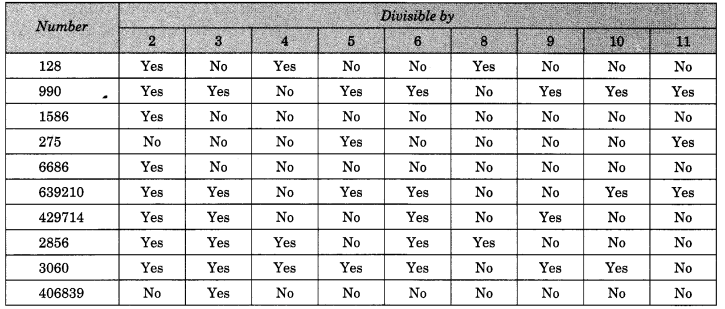## Ex 3.3 Question 2.

Using divisibility tests, determine which of the following numbers are divisible by 4; by 8.
(a) 572
(b) 726352
(c) 5500
(d) 6000
(e) 12159
(f) 14560
(g) 21084
(j) 2150
(h) 31795072
(i) 1700
Solution:

(a) 572
Divisible by 4 as it’s last two digits are divisible by 4.Remainder 0. Hence, 572 is divisible by 4.

Not divisible by 8 as it’s last three digits are not divisible by 8.Remainder 4. Hence, 572 is not divisible by 8.

(b)  726352

Divisible by 4 as it’s last two digits are divisible by 4.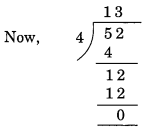Remainder = 0.
Hence, 726352 is divisible by 4.

Divisible by 8 as it’s last two digits are divisible by 8.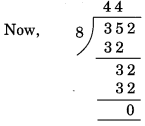Remainder = 0.
Hence, 726352 is divisible by 8.

(c)  5500
Divisible by 4 as it’s last two digits are divisible by 4.

Not divisible by 8 as it’s last three digits are not divisible by 8.Remainder = 4. Hence, 5500 is not divisible by 8.

(d)6000
Divisible by 4 as it’s last two digits are divisible by 4.
Hence, 6000 is divisible by 4.

Divisible by 8 as it’s last two digits are divisible by 8.
Hence, 6000 is divisible by 8.

(e)12159
Divisible by 4 as it’s last two digits are divisible by 4.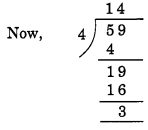Remainder = 3.
Hence, 12159 is divisible by 4.

Not divisible by 8 as it’s last three digits are not divisible by 8.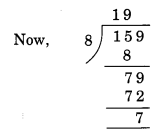Remainder = 7. Hence, 12159 is not divisible by 8.

(f)14560
Divisible by 4 as it’s last two digits are divisible by 4.Remainder = 0.
Hence, 14560 is divisible by 4.

Divisible by 8 as it’s last two digits are divisible by 8.

(g) 21084
Divisible by 4 as it’s last two digits are divisible by 4.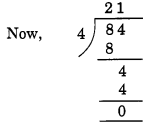Remainder = 0. Hence, 21084 is divisible by 4.

Not divisible by 8 as it’s last three digits are not divisible by 8.Remainder = 4. Hence, 21084 is not divisible by 8.

(h) 31795072
Divisible by 4 as it’s last two digits are divisible by 4.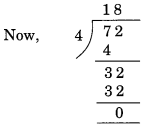Remainder = 0. Hence, 31795072 is divisible by 4.

Divisible by 8 as it’s last two digits are divisible by 8.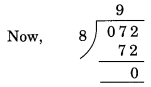Remainder = 0. Hence, 31795072 is divisible by 8.

(i) 1700
Divisible by 4 as it’s last two digits are divisible by 4.
Not divisible by 8 as it’s last three digits are not divisible by 8.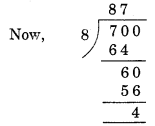Remainder = 4. Hence, 1700 is not divisible by 8.
(j) 2150
Divisible by 4 as it’s last two digits are divisible by 4.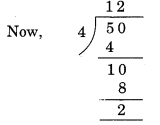Remainder = 2. Hence, 2150 is not divisible by 4.

Not divisible by 8 as it’s last three digits are not divisible by 8.Remainder = 6. Hence, 2150 is not divisible by 8.

## Ex 3.3 Question 3.

Using divisibility tests, determine which of the following numbers are divisible by 6:
(a) 297144
(b) 1258
(c) 4335
(d) 61233
(e) 901352
(f) 438750
(g) 1790184
(h) 12583
(i) 639210
(j) 17852
Solution:

(a) 297144
Divisible by 2 as it’s unit place is an even number.
The sum of all the digits of 297144 = 2 + 9 + 7 + 1 + 4 + 4 = 27
which is divisible by 3.
Hence, the given number 297144 is divisible by 6.

(b) 1258
Divisible by 2 as it’s unit place is an even number.
The sum of all digits of 1258 = l + 2 + 5 + 8 = 16

which is not divisible by 3.
Hence, the given number 1258 is not divisible by 6.

(c) 4335
Not divisible by 2 as it’s unit place is not an even number.
The sum of all the digits of 4335 = 4 + 3 + 3 + 5 = 15 which is divisible by 3.
Since the number 4335 is not divisible by both 2 and 3 therefore, it is not divisible by 6.

(d) 61233
Not divisible by 2 as it’s unit place is not an even number.
The sum of the digits of the given number 61233 = 6 + 1 + 2 + 3 + 3 = 15 which is divisible by 3.
Since the number 61233 is not divisible by both 2 and 3, it is not divisible by 6.

(e) 901352
Divisible by 2 as it’s unit place is an even number.
The sum of all the digits of the given number 901352 = 9 + 0 + 1 + 3 + 5 + 2 = 20 which is not divisible by 3.
Since the number 901352 is not divisible by both 2 and 3 hence, it is not divisible by 6.

(f) 438750
Divisible by 2 as it’s unit place is an even number.
The sum of all the digits of the given number 438750
=4 + 3 + 8 + 7 + 5 + 0 = 27 which is divisible by 3.
Hence, the number 438750 is divisible by 6.

(g)1790184
Divisible by 2 as it’s unit place is an even number.
The sum of all the digits of the given number 1790184
= 1 + 7 + 9 + 0 + 1 + 8 + 4 = 30 which is divisible by 3.
Hence, the number 1790184 is divisible by 6.

(h) 12583
Not divisible by 2 as it’s unit place is not an even number.
Sum of all the digits of the given number 12583
= 1 + 2 + 5 + 8 + 3 = 19
which is not divisible by 3.
Hence, the number 12583 is not divisible by 6.

(i) 639210
Divisible by 2 as it’s unit place is an even number.
The sum of all the digits of the given number 639210
= 6 + 3 + 9 + 2 + 1 + 0 = 21 which is divisible by 3.
Hence, the number 639210 is divisible by 6.

(j) 17852
Divisible by 2 as it’s unit place is an even number.
The sum of all the digits of the given number 17852
= 1 + 7 + 8 + 5 + 2 = 23 which is not divisible by 3.
Hence, the number 17852 is not divisible by 6.

## Ex 3.2 Question 4.

Using divisibility tests, determine which of the following numbers are divisible by 11:
(a) 5445
(b) 10824
(c) 7138965
(d) 70169308
(e) 10000001.

(a) 5445
Sum of the digits at odd places = 5 + 4 = 9
Sum of the digits at even places = 4 + 5 = 9
Difference of both sums = 9 – 9 = 0
Hence, the number is divisible by 11.

(b) 10824
Sum of the digits at odd places = 4 + 8 + 1 = 13
Sum of the digits at even places = 2 + 0 = 2
Difference of both sums= 13 – 2 = 11
Hence, the number is divisible by 11.

(c) 7138965
Sum of the digits at odd places = 5 + 9 + 3 + 7 = 24
Sum of the digits at even places = 6 + 8 + 1 = 15
Difference of both sums = 24 – 15 = 9
Hence, the number is not divisible by 11.

(d) 70169308
Sum of all the digits at odd places = 8 + 3 + 6 + 0 = 17
Sum of all the digits at even places = 0 + 9 + 1 + 7 = 17
Difference of both sums = 17-17 = 0
Hence, the number is divisible by 11.

(e) 10000001
Sum of all the digits at odd places = 1 + 0 + 0 + 0 = 1
Sum of all the digits at even places = 0 + 0 + 0 + 1 = 1
Difference of both sums = 1 – 1 = 0
Hence, the number is divisible by 11.

## Ex 3.2 Question 5.

Write the smallest digit and the greatest digit in the blank space of each of the following numbers so that the number formed is divisible by 3.
(a) ____ 6724
(b) 4765 ____ 2
Solution:
We know that number is divisible by 3 if the sum of all the digits of the number is also divisible by 3.
(a) ___ 6724
Sum of the digits = 4 + 2 + 7 + 6 = 19
The smallest digit  = 2
Then the sum = 19 + 2 = 21 which is divisible by 3.
The largest digit  = 8
Then, the sum = 19 + 8 = 27 which is divisible by 3
Hence, the required digits are 2 and 8.

(b) 4765 ____ 2.
Sum of digits = 2 + 5 + 6 + 7 + 4 = 24
The smallest digits  = 0
Then, sum = 24 + 0 = 24
which is divisible by 3.
The largest digit  = 9.
Then, the sum = 24 + 9 = 33 which is divisible by 3.
Hence, the required digits are 0 and 9.

## Ex 3.2  Question 6.

Write a digit in the blank space of each of the following numbers so that the numbers formed is divisible by 11.
(a) 92 ___ 389
(b) 8 ___ 9484
Solution:
(a) 92 ___ 389
Sum of the digits at odd places = 9 + 3 + 2 = 14
Sum of the digits at even places = 8 + ( ) + 9 = 17
Difference = 17 + ( ) – 14 = () + 3
For the given number to be divisible by 11
( ) + 3 = 11
∴ ( ) = 11 – 3 = 8
So, the missing digit = 8
Hence, the required number is 928389.

(b) 8 ___ 9484
Sum of the digits at odd places = 4 + 4 + ( ) = 8 + ( )
Sum of the digits at even places = 8 + 9 + 8 = 25
∴ Difference = 25 – [8 + ( )]
= 25 – 8 – ( ) = 17 – ( )
For the given number to be divisible by 11
17 – 0 = 11
∴ 17 – 11 = 6
So, the missing digit = 6
Hence, the required number = 869484.

error: Content is protected !!